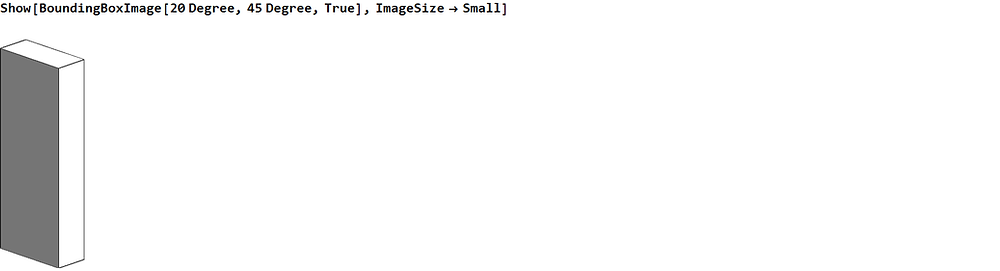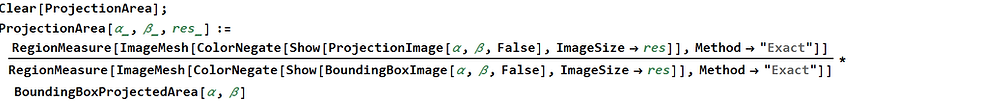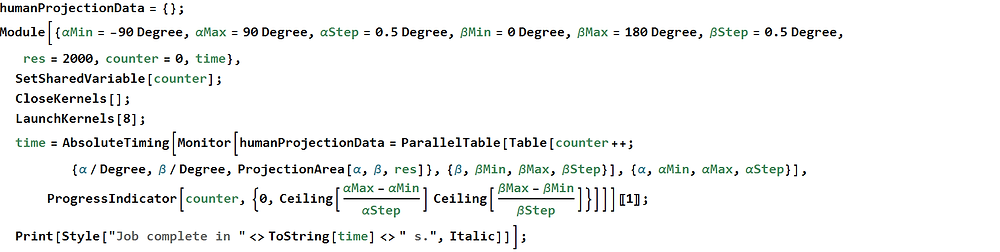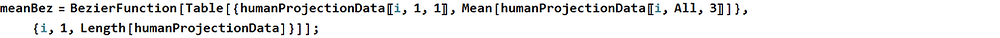# The Human Body: 3D Image Projection in Mathematica

In my blog post titled "Can bullets fired into the air kill?", we had examined the physics of bullet flight, and the possibility of rifle bullets causing injuries or death after being fired into the sky—and in the last section we had attempted to calculate an approximate probability of lethal impact. To do that, it was necessary to determine the areal density of the human population here in Singapore, as seen from the projectile's perspective as it descends.

We had said then that such a problem was equivalent to calculating the two-dimensional projected area of the three-dimensional human body, give or take some trivial arithmetic to convert between absolute area and areal density. And we had also said that it was perfectly possible to do this computationally. In this post we would see exactly how it was done.

The Human Body in Mathematica

It might come as a surprise, but yes, Mathematica does in fact have rather extensive support for computation on the human body. The idea is that it can be used to aid in biological and medical research—and to that end, the latest versions of Mathematica has built-in marching-cube algorithms for processing three-dimensional MRI/CT scan data, native support for discrete three-dimensional images, and even functions for downloading anatomical data from Wolfram database. That last feature is what we'll be utilizing in this post.

Let us first experiment with the function AnatomyPlot3D. The input of AnatomyPlot3D can be given as natural-language dictation of the parts of the human body to plot. This is the same technology that allows Wolfram Alpha to interpret free-form natural language expressions from users. Because of this added flexibility, and because Mathematica would need to download the anatomical data from the Wolfram database, Internet connectivity is required for this function to run.Above we have plotted out the anatomical structure of the human hand—and we can see that Mathematica automatically interweaves the numerous layers of human tissue. The plot is given as a styled Graphics3D object, which we can manipulate interactively. Another example on this—we can dictate that it plot our skeletal structure.We wish to calculate the projection of a human body, and that means we require an outer shell of the body, sufficient to represent our exterior surface. In other words, we require the skin of a human. All other internal anatomy is irrelevant for our purposes.At this point we might have a question in mind—how are the dimensions of the plotted body decided upon? There is, of course, natural variation in the height and width of a body, as well as fat distribution and muscle tone; surely this single plot cannot represent the diversity amongst our population. The answer is that the plotted body corresponds to the statistical average of our human population.

Now we can begin our computational adventure. It would be nice to have a 3D model to work with, rather than a plot—perhaps a MeshRegion, so that we can manipulate and transform its geometry if we need to. It is also computationally cheaper to render a MeshRegion with minimal styling than to call AnatomyPlot3D, because we can skip the complex shading and colouring.

I had previously written blog posts on the manipulation of MeshRegion objects, the most recent being "Tank Rover: Animations" concerning the creation of animations from CAD models, and we can see for ourselves that MeshRegions are an exceedingly flexible computational tool. Indeed there is a built-in function to import a MeshRegion of human anatomy—AnatomyData.Above we have named the imported MeshRegion humanReg, short for human region. Now we can proceed to think about the ways to compute a projection of this model. The general idea is the same—we render in three dimensions the human body from our desired view point, flatten it to two dimensions and compute its area. Then we calculate, through geometrical considerations or otherwise, the effective area of the flattened projection.

Projection Mathematics and Computation

We hadn't really explained why calculating the population areal density is equivalent to calculating a two-dimensional projection of the human body. To see the connection, we need first see exactly what a projection means. Let us simplify things a little and get rid of one spatial dimension, and draw the scenario out.A projection of an object from some viewpoint is the flat image of that object as seen by an optical device placed at that viewpoint; and in order for such an image to be perceived, light rays from the object must reach the device. We say that the image is flat, because it is without depth information. In the diagram above these light rays are presented in red. We have taken the viewpoint to be at an angle α to the horizontal, placed infinitely far away—in other words, we're seeking an orthographic projection—and therefore our light rays are parallel to each other.

The reason for placing our viewpoint infinitely far away has got to do with our interest in the population areal density. In the context of our probabilistic equations, and as implied by the term density, we are concerned with an average case. We are not so much interested in how a projectile would interact with a single human placed at some relative angle; rather, we are interested in how countlessly numerous projectiles, all with the same velocity and angle of attack, would interact with countlessly numerous human targets, each oriented in a random direction with respect to the incoming projectiles.

We talk about an average case—but what is to be averaged? What is smeared out, in our averaging process? The answer is that we are smearing out the position dependence of the projectile-target interactions. Because we cannot possibly know the exact position of every individual in Singapore, we cannot do an exact simulation of projectile impacts upon our human population. Further, we do not know where the projectile is to be fired from; and for the sake of generality, we ought not to simply assume a place. The logical way is to look at the average case, assuming equal probability of the projectile being fired at any position in Singapore, and equal probability of any individual to be at any place at any time. We, in essence, smear out the positions of the firer and the human population, to be uniformly distributed across the whole of Singapore.

This is the reason for there being countlessly numerous potential projectiles, each with identical velocity and angle of attack but different positions, and there being countlessly numerous potential targets, each with identical average body form but different positions and orientations. There is a direct congruence between the parallel light rays that strike our human figure above, and the countlessly numerous projectiles that approach any individual target. And therefore calculating the population areal density can be reduced to calculating a two-dimensional orthographic projection of the human body.The viewpoint for the projection can be characterized by an elevation angle α and an azimuthal angle β, corresponding to the incoming trajectory angle of the projectile and its azimuthal angle, respectively. Eventually we would need to average the calculated projection area over β, consistent with our random-orientation heuristic as explained.

First we have to figure out how to render a three-dimensional graphic of the human body from a particular viewpoint. The viewpoint P of a rendering can be quite trivially set by specifying the ViewPoint option in Show; and to make it orthographic, we simply scale the viewpoint by a large amount, so that it is orders of magnitude larger than the dimensions of the human body. The default units of measure of human anatomy in Mathematica is millimeters, so this scaling should preferably be much larger than a thousand.We can define a function, ProjectionImage, to perform this rendering for us. The code is presented below.We have taken the scaling factor to be a million; and we have also introduced a lighting boolean argument. If, during the calling of this function, we set lighting to be true, then the rendered human body would have the default MeshRegion shading and styling; else, the rendered human body would be styled completely black against a white background. This high-contrast, computationally cheap mode would be useful later on, as we'll see.

We have also set PlotRangePadding to be zero, and have specified the PlotRange to be exactly the bounding dimensions of the human model. This is so that no extra space on the rendered image is wasted on non-human regions. On one hand, this is critical for the functioning of a particular method, as we'll explain later; and on the other, this reduces the computational cost of our function further.

Running this function gives us the results that we expect.

Now we need to flatten the rendered graphics to a two-dimensional one, and calculate its area. There are two ways of doing this: the first is to apply ImageMesh straight on the rendered graphics, which allows us to flatten it and calculate its area in one go; and the second is to rasterize the graphics, and then do a pixel count. We will present both of these methods in separate subsections below, for the sake of completeness; but in truth the first method is significantly faster, and so for all practical purposes the second is obsolete.

ImageMesh Method

ImageMesh is a built-in function that takes in an image and produces a BoundaryMeshRegion of its foreground, as determined through contrast and morphological filtering. To make our black-on-white human graphics show up as the foreground, we perform colour negation, such that it is now white-on-black, before passing it into ImageMesh. We can test it out as follows.We have explicitly requested for the Exact method, because it is for some reason much faster than the Marching Cubes and Dual Marching Cubes alternatives. And as a bonus it is supposedly more accurate than the alternatives, as its name implies. Following this, we can use RegionMeasure to obtain the area of the generated BoundaryMeshRegion.We now need to answer a question—in what unit of measure is this calculated area presented in? We know the default unit for human anatomy in Mathematica is millimeters, and we have done no explicit geometric transformations on the image, so we would not expect this to change. However, we have implicitly rasterized our three-dimensional graphics to a two-dimensional image by calling ImageMesh; and this ruins our unit of measure. The area returned by ImageMesh has now become arbitrary, proportional to the dimensions of the rasterized graphics.

We can, of course, scale the unit of measure back to millimeters by computing the bounds of the rasterized graphics and performing some trigonometry. But we need not go through all this trouble. An easier way is to use a reference object whose area is known—and a convenient object to use is the bounding box of our human model. We define a function, BoundingBoxImage, in a completely identical manner to the way we defined ProjectionImage.Calling this function, we see that it renders a graphic of a cuboid bounding box that exactly encloses our human figure. As before, setting lighting to false would render a black-on-white shadow image.Inputting the same angles into ProjectionImage and BoundingBoxImage would produce a pair of corresponding images, cropped in exactly the same way and rendered from exactly the same viewpoint; and the key is that we are able to calculate the visible area of the bounding box analytically. Denoting the dimensions of the bounding box Δx, Δy and Δz in the three directions respectively, we can write the following formula:

Using this, we can write the following expression for the actual projected area of our human body, where the areas in arbitrary units as computed by RegionMeasure are presented with star superscripts.

We have our mathematical basis; now onto the programmatic aspects. We define the following auxiliary function, BoundingBoxProjectedArea, to calculate the projected area of the bounding box analytically. Note the shorthand that we have used for mapping functions onto lists.And we also write the primary function ProjectionArea to calculate the projected area of our human target.Above we have introduced an argument named res, short for resolution, that determines the dimensions of the rasterized images processed by ImageMesh. The greater this resolution, the more accurate the determination of the BoundaryMeshRegion would be, and this would in turn propagates towards more accurate area calculations; but this is at an expense of computational speed. We roughly expect the computation time to scale quadratically with image size, so a large value of res might not be practically feasible. Through trial-and-error, a res value between 2000 and 3000 seems to be ideal.

We are now able to compute the projected area of our human figure by calling a single function. All that is left is to iterate over different values of α and β. In order to leverage on parallelization to speed things up, we use ParallelTable for iteration; and to monitor the progress of our computation, which would span multiple hours, we use Monitor coupled with a ProgressIndicator. As usual, we define a counter variable to be shared across all kernels, and use this to feed the ProgressIndicator.Notice that we evaluate only a 180-degree range of α and β in our loop. This is because of the intrinsic lateral symmetry of the human figure—evaluating past these 180-degree domains would be equivalent to reflecting the data that we had already computed. Exploiting this symmetry allows us to save time.

And we're essentially done. All we need to do is let the code run for a few—perhaps closer to a dozen—hours. Afterwards one may choose to export the data to a file for safekeeping, or proceed straight on to post-processing.

Pixel-Counting Method

As mentioned previously, there are two methods for carrying out the area calculations, The first is to use ImageMesh, which we have explained above. The second is to explicitly rasterize the images, and then to do a pixel count of all black pixels—we will examine this alternative in this subsection.

We can modify our equation for a pixel-counting approach, with the pixel counts denoted by N. This is of identical form to our area equation earlier.

As before, we utilize ProjectionImage and BoundingBoxImage to generate the three-dimensional graphics; but this time we enclose them in Rasterize, with image dimensions specified by argument res. As before, because the computational cost scales quadratically with image size, it might not be feasible to increase res beyond a certain limit. We also use Binarize to convert our images to single-channel black-and-white, to speed up pixel counting; and we use ImageMeasurements to do a pixel count on black pixels.The reason why ImageMeasurements can be conveniently utilized here is that in a binary image, white pixels are represented with value 1, and black pixels with value 0. We dictate that ImageMeasurements is to return the total value of the image, which, as a result of the binary representation of pixel values, would correspond to the total number of white pixels. We desire a count on black pixels, since our images are black-on-white, and therefore we negate the image right before passing it to ImageMeasurements.

Back to the topic on hand. We need only replace the ProjectionArea function as shown above; there is no need for us to touch our iteration loop. We can run our ParallelTable as before, and wait for our results.

There is one thing we should know, however. While these two methods give very similar results, with discretization error accounting for whatever minor discrepancies, they differ considerably in performance. Let us give an example.We can see that the ImageMesh method outperforms the pixel-counting method by about three times. This can be the difference between 12 hours of computation, and a day and a half. The reason for this performance gap lie in the explicit rasterization and the pixel-counting, which are much slower than the implicit rasterization and construction of boundary meshes. For practical purposes, it seems the ImageMesh method is always preferable.

Data Post-Processing

Now that we have our data, we can proceed to post-processing and visualization. In the context of our "Can bullets fired into the air kill?" blog post, we desire the β-averaged projected area, as a function of α. Perhaps before carrying out the averaging, we would like to see how the projected area varies with β.

Because of the presence of discretization errors, it would be helpful to smooth the data. There are many ways to do this, such as using a low-pass filter, or by doing polynomial fits. In our case we opt to smooth the data using Bezier functions. We first apply a small-radius moving average on our data to get rid of large jumps, and then we evaluate a Bezier function, using the datapoints as anchors.Following this, we can plot our smoothed projected area against β using ParametricPlot.And these are the graphs that we obtain, for positive and negative α on the left and right respectively. Using the code above, one is free to change the values of α to plot, by specifying them in the internal list.

And now onto the averaging process. Mathematically it is simply a uniformly-weighted integral average, as follows.

Programatically, we can get rid of the integral altogether and use a discrete sum, because our data is discrete in the first place. Better yet, Mathematica has a built-in function Mean, to calculate the arithmetic mean of a list. As before, to smooth the data, we construct a Bezier function with our data as anchor points.And again, we can use ParametricPlot to graph our Bezier function. This leads us to the following plot of our final, post-processed data.And that's it, we're done! Calculating the population areal density from projected area is already presented in my "Can bullets fired into the air kill?" blog post, so I shall not repeat the explanation here. Please jump over to the blog post if you would like to find out more.

If not, till next time, goodbye!